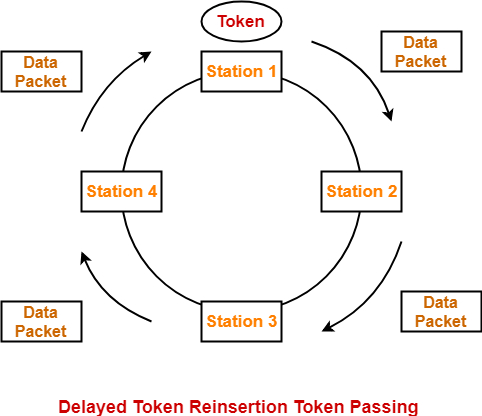# What is early token release and delayed token release?

Token ring is a physical unidirectional ring, where the systems are connected to the ring through some interface.

In the token ring a special frame is called a token which is always circulated on the ring. If a system has to transmit then it needs to acquire the token without a token no system can transmit. Only one system can transmit at a time. Hence it is collision free protocol.

The token ring source is responsible for removing the frame from the ring. In normal token ring operation, a station sending information holds the token until the sending data circles the entire ring.

After the sending station strips the data from the ring, it then issues a free token. The system regenerates the token after arrival of frame or after transmission of frame.

#### Types of Token rings

We divide token ring into two types which are as follows −

## Early Token release

System immediately regenerates the token after frame transmission without waiting for arrival. In delay token release system generates the token only after frame arrival.

The efficiency of early token release is as follows −

• When only one system wants to transmit.
• Every system wants to transmit.

Ring latency − The ring latency or propagation delay is a time taken by a single bit to travel a complete ring. We assume systems are equidistant.

If ring latency is ‘R’ and total number of systems is ‘N’ then time taken by a single bit to travel between two intermediate stations = R/N.

Let us see the efficiency of early token when only one wants system wants to transmit −

Total time taken = tx+ txt+ tp

Where tx= frame transmission time and

t xt = token transmission time.

$\mathrm{\eta\:=\:t_x/(t_x\:+\:t_{xt}\:+\:t_p)}$

If length of token is not given txt is negligible. Token transmission is overhead then it is not a useful protocol. If there is some kind of delay then we will add that token ring as it is with overhead.

Where the frame is stored there we have to consider transmission time of the system and where it is not stored we don’t have to consider.

Let us see the efficiency when everyone wants to transmit −

$\mathrm{\eta\:=\:t_x/(t_x\:+\:t_{xt}\:+\:t_p/N)}$

## Delayed token release

In delayed token release the token keeps holding until the last bit of the data packet transmitted, after the complete revolution of the ring and comes back to it.

Whenever a station acquires the token, then it does the following −

• It transmits the data packet.

• The token will be held until the data packet reaches back to it.

• Whenever the data packet reaches, it discards the packet because its journey is completed.

• Finally it releases the token.

Given below is the diagram of delayed token release −The efficiency of delayed token when only one system wants to transmit −

Total time taken = $\mathrm{t_x+t_p\:+\:t_{xt}\:+\:t_p}$

tx+ tp=frame transmission and traversal.

txt+ tp= token transmission traversal.

The efficiency of delayed token when everyone wants to transmit −

$\eta\:=\:t_x/(t_x\:+\:t_{p}\:+\:t_{xt}\:+\:t_p/N)$